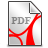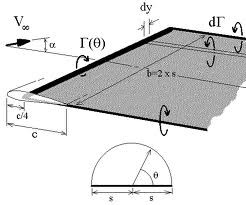# the Prandtl hyper-singular integral operator

enabling an integral equation with double layer potential defining an alternative H(1/2) energy Hilbert space framework

We propose to apply the Prandtl operator theory and the related hyper-singular integral equation with double layer potential (solving the Neumann Problem) alternatively to the Laplacian operator to define the alternative energy Hilbert space H(1/2).Braun K., The Prandtl (hypersingular) integral equation with double layer potential and the exterior Neumann problem_1.pdf (315.97KB)Braun K., The Prandtl (hypersingular) integral equation with double layer potential and the exterior Neumann problem_1.pdf (315.97KB)

Some related topics

The Biot-Savart law describes the magnetic field force (Maxwell), calculated from a electric current density (or the flow/current field (Navier), calculated from a vortex field). The vortex field is "generated" by:

- the "truly" potential stream, which is vortex-free per definition

- artificially "generating" boundary layer vortex forces.

The "root cause" of "uplift forces" in the Biot-Savart law is captured by the circulation constant.

The "vortex line" was introduced by L. Prandtl: it is a mathematical arte-fact to "explain" its induced "circuit/ vortex force", which "generates"/ is the root cause of the "uplift force":

- the (vortex density)(u(x)) is given by the rotation of u(x)  = rot(u)(x);

- the (vortex force)(x) is given by the (normal vector) n times (rot(u)(x)).

We propose to represent the vortex force by the components of a corresponding Plemelj-Stieltjes potential, which requires less regularity assumptions than current standard model. In fact, the Prandtl model already anticipated a sort of "energy" inner product being appropriate to apply it in the context of double boundary layers, mathematical treated in the framework of complementary variational methods.

From (GlH) we summarize (not quote) the following (1.2):

"The development of an airfoil lift theory goes back to Kutta, Joukowski (n=2) and Lancester, Prandtl (n=3). The theory delivers results, which matches quite good with experimental results. However it remains the difficulty to explain the origin of the circulation, based on the assumption that the "fluid" (air) is considered as homogeneous medium, whereby in its equilibrium state no shear stresses can be transmitted. The viscosity of air can be neglected in many cases. Considering air as an incompressible, frictonless medium then a body, which moves through this ideal "liquid" does not experience any resulting forces (Helmholtz, Kirchhoff). This miss match between theoretical assumptions and experimental results manifests in the question of the root cause of the circulation."

From Trefftz R., §1, we quote: "(man) erhält einen Auftrieb, wenn man in die Fläche einen Wirbelfaden legt, der eine Zirkulation um den Flügel hervorruft, ... mathematisch gesprochen, wenn man eine Mehrdeutigkeit des Potentials in den die Tragfläche umgebenden zweifach zusammenhängenden Raum zulässt. ...."Gamma" (ist) die Zirkulations-/Wirbelstärke ("vortex force") dieses Wirbelfadens";

Conceptually the above means, that a priori there is given a (rotation-/curl-free) potential and (only!) a posteriori there is added/introduced a mathematical arte-fact (a vortex line) to "generate" the obviously existing uplift force via a circuit force. The same concept is followed to describe the resistance force.

In this context we refer to the idea of J. Plemelj about a "normal vector" being defined only by values of the interior domain (!). We note that the circulation measures how strong the liquid "circulates" along a given curve K. The normal component (!) of the rotation of a vector (velocity) field v can be physically interpreted as limit of the circulation per area element. The line integral of the velocity of a liquid is called ""flow" or "current"; along a closed curve it´s called "circulation".  A flow w/o a "circulation" is always curl-free. The vortex force (vorticity) of a vortex-threats has along its whole length and at any point in time the same value.

In the plane, stationary, curl-free situation there is a complete analogue between modeling an electric and an aero-dynamical potential. however, the 3-D-Maxwell equations require the mathematical arte-fact of the "Verschiebestrom" (displacement current).

Remark 1

In the paper of Zahang & Chen a new integral (auxiliary) equation concerning the thin airfoil problem is proposed. The unknown function represents vorticity along the thin airfoil. The equation has logarithm kernel (which is of Symm operator type), which is a weaker singularity than the "standard" one (which is of Hilbert operator type). After determination of the vorticity the lift forces and moment acting on the thin airfoil (described by the original equation) can be solved according to the Blasius formula.

Remark 2

For a linkage between the author's paper ("A new ground state energy model", "An alternative quantization of H=xp") and "problems in aerodynamics" we refer to (LiI) and (LiI1) chapter 11, "Problems in Aerodynamics", section "Non-Traditional Approach, Remark 11.1.4, 11.1.5".

Remark 3

For background information and notation of remark 4 we note:

let X=X(x,y) denote the potential and Y=Y(x,y) denote the flow function of the complex value field v=v(x,y). The complex valued, holomorph function

F(z)=F(x+iy)=X(x,y)+Y(x.y)

is called the complex valued flow function. The function X is the conjugate complex harmonic function of Y. From a known complex potential function F the velocity field v and the pressure distribution of the flow can be calculated:

- the velocity field v can be derived by differentiating the flow function F and then taking the conjugate complex function from this result

- the flow N and the circulation G are the real and the imaginary part of the line K integral over the conjugate complex velocity field v, whereby K is a positive oriented, closed, double point free and smooth curve. The residuen theorem gives:

G+iN=2*(pi)*i*a(-1)   .

Flow stream lines are curves, where the flow velocity vector is tangent to that curve at any point on the curve.

Remark 4

The case n=2 is supported by the full power of the complex analysis. Nevertheless the conceptual issues with respect to the singularities at stagnation points or corners for "ideal" fluids are still not solved.

A partial Laplace differential equation is derived from the potential function and the flow function to get the analytical solution of the 2D flow. The conceptual challenge is, that the model requires, that vortex area is per definition a liquid and that the pressure on the vortex area is continuous, but there is obviously a velocity "jump" e.g. in case of an edge (Prandtl L., Über die Entstehung von Wirbeln ...).

In the paper of Blum H./Dobrowolski M. singular functions of the Laplacian (potential flow) problem in the trial space are used in combination with kernel functions of the adjoint problem (with respect to the Hilbert space L(2)) to define an appropriate "quasi-optimal" FEM approximation solution. The result is based on corresponding shift theorems for elliptic problems with domains with corners (Kondrat'ev, Grisvard, Maz'ja-Plamenevshij).